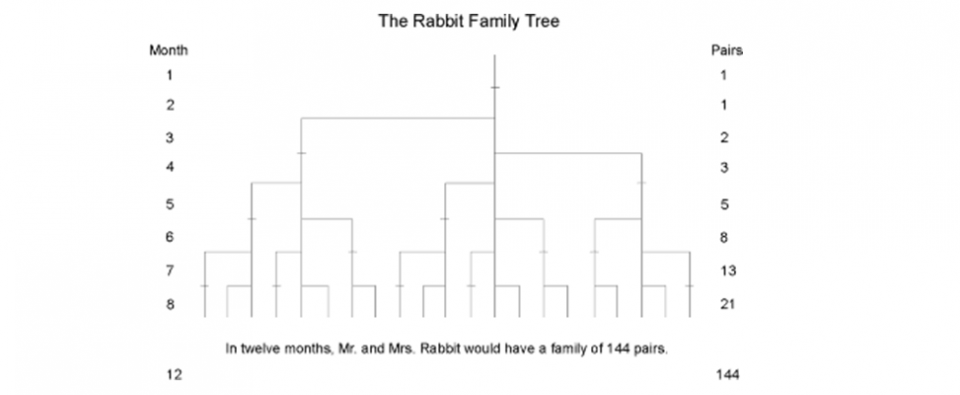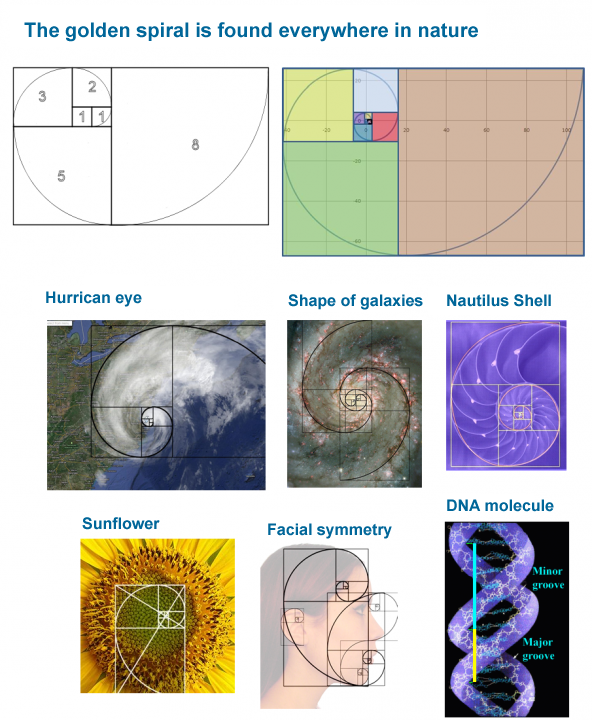### measuring processes of additive growth

Market cycles of similar size – or degree – share certain mathematical properties widely found throughout nature.

### HISTORICAL BACKGROUND

The Fibonacci sequence of numbers was discovered (actually rediscovered) by Leonardo Fibonacci da Pisa, a thirteenth century mathematician. When R.N. Elliott wrote Nature’s Law: the secrets of the universe, he referred specifically to the Fibonacci sequence as the mathematical basis for the Wave Principle. It is sufficient to state at this point that the stock market has a propensity to demonstrate a form that can be aligned with the form present in the Fibonacci sequence. (For a further discussion of the mathematics behind the Wave Principle, see “Mathematical Basis of Wave Theory,” by Walter E. White, in New Classics Library’s book.)

### THE FIBONACCI SEQUENCE

In the early 1200s, Leonardo Fibonacci of Pisa, Italy published his famous Liber Abacci (Book of Calculation) which introduced to Europe one of the greatest mathematical discoveries of all time, namely the decimal system, including the positioning of zero as the first digit in the notation of the number scale. This system, which included the familiar symbols 0, 1, 2, 3, 4, 5, 6, 7, 8 and 9, became known as the Hindu-Arabic system, which is now universally used.

In Liber Abacci, a problem is posed that gives rise to the sequence of numbers 1, 1, 2, 3, 5, 8, 13, 21, 34, 55, 89, 144, and so on to infinity, known today as the Fibonacci sequence. The problem is this:

How many pairs of rabbits placed in an enclosed area can be produced in a single year from one pair of rabbits if each pair gives birth to a new pair each month starting with the second month?

In arriving at the solution, we find that each pair, including the first pair, needs a month’s time to mature, but once in production, begets a new pair each month. The number of pairs is the same at the beginning of each of the first two months, so the sequence is 1, 1. This first pair finally doubles its number during the second month, so that there are two pairs at the beginning of the third month. Of these, the older pair begets a third pair the following month so that at the beginning of the fourth month, the sequence expands 1, 1, 2, 3. Of these three, the two older pairs reproduce, but not the youngest pair, so the number of rabbit pairs expands to five. The next month, three pairs reproduce so the sequence expands to 1, 1, 2, 3, 5, 8 and so forth. The Rabbit family grows with logarithmic acceleration. Continue the sequence for a few years and the numbers become astronomical. In 100 months, for instance, we would have to contend with 354,224,848,179,261,915,075 pairs of rabbits.

The diagram below illustrates the concept:The Fibonacci sequence resulting from the rabbit problem has many interesting properties and reflects several interesting relationships among its components:

• Each term equals the sum of the preceding two terms;

• As the series goes to infinity, the ratio between a term and the next higher term tends to 0.618.

In other words as n tends to ∞, the Xn-1/Xn ratio tends to 0.618;

• As the series goes to infinity, the ratio between a term and the second next higher term tends to 0.382.

In other words as n tends to ∞, the Xn-2/Xn ratio tends to 0.382 (or 1 – 0.618);

• As the series goes to infinity, the ratio between a term and the next lower term tends to 1.618.

In other words as n tends to ∞, the Xn/Xn-1 ratio tends to 1.618 ( or 1 + 0.618 );

• In math, 0.618 is the only real number r for which the property 1/(r) = 1 + r holds true.

In other words, 1/(0.618) = 1 + 0.618;

• Because of that, 0.618 is the only real number for which the relationship (r)n+1 = 1 – (r)n holds true for any natural number n.

In other words (0.618)n+1 = 1 – (0.618)n;

Market movements of similar degree tend to be connected at such fibonacci ratios. Something really intriguing is that what seems to matter most is ratio not actual nominal sizes measured and this adds evidence to the idea that the Fibonacci ratios are in fact a mathematical property of all series that represent processes of additive growth.

Very interestingly, it can be demonstrated that for any series starting with any two terms X1 and X2 for which subsequent terms can be formed as the sum of the preceding two, i.e. Xn = Xn-1 + Xn-2 , the following properties hold true as n tends to ∞:

• the Xn/Xn-1 ratio tends to 1.618 (or 1 + 0.618 ) ;

• the Xn-1/Xn ratio tends to 0.618 ;

• the Xn-2/Xn ratio tends to 0.382 or (1 – 0.618).

It can be seen in the pictures below that the Fibonacci series can form the basis of the so-called golden spiral (as shown in the first two diagrams). A remarkable trait is that the golden spiral is the mathematical law that seems to apply to virtually all processes of additive growth found in nature, from structures as small as the DNA molecule to as large as the shape of the galaxies and everything else in between (including the eyes of hurricanes, the horns of animals, the shapes of sea shells or the cones and flowers of plants).##### The decoupling
27 April, 2020
0

Correlation patterns at the asset class level stem from two sources, that is discounting factors and the way sectors and industry groups absorb higher or lower interest rates based on […]##### Shivers on the back ….
02 April, 2020
0

The wave principle is unique in the perspective it can give on market trends. Many technicians find themselves confused with Elliott and present as criticism the subjective nature of wave […]